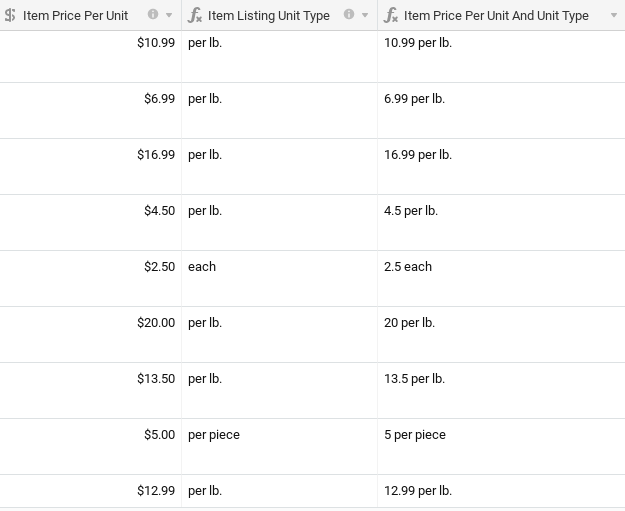# List Field Formatting Options

I would love to get some more formatting options for text fields in a table list. I am using Softr to list available rental properties. My list should be able to format or include dollar signs for prices, control decimal places for numbers, etc…

2 Likes

As a workaround in the meantime, if you format the rent amount as a text field in airtable and include the dollar sign, think that works , or you could use a formula field to format. But yes I agree would be helpful to have formatting capability in Softr!

1 Like

I do have it formatted properly in Airtable as a formula. The formatting from Airtable is not being maintained in Softr.

I agree and 2nd this request.

Hi. Any idea how to keep the zeros using an airtable formula when converting a number in a currency field? For instance when you have \$5.00 in a currency field and you want to convert it to “\$5.00 per lb” using a formula, the output is “\$5 per lb”. Or worse, if the currency field is “\$5.50” the formula field output is “\$5.5 per lb”.

Hi @Ben ,

I think this formula solves your issue.

`````` CONCATENATE(IF(
LEFT({NumberValue}&'',1,1)='-',
'-',
'')&'\$'&
IF(
ABS(VALUE({NumberValue}&''))>=1000000,
INT(ABS(VALUE({NumberValue}&''))/1000000)&','&
IF(
INT(MOD(VALUE({NumberValue}&''),1000000)/1000)<100,
REPT('0',3-LEN(INT(MOD(VALUE({NumberValue}&''),1000000)/1000)&'')),
'')&INT(MOD(VALUE({NumberValue}&''),1000000)/1000)&','&
IF(
INT(MOD(VALUE({NumberValue}&''),1000))<100,
REPT('0',3-LEN(INT(MOD(VALUE({NumberValue}&''),1000))&'')),
'')&INT(MOD(VALUE({NumberValue}&''),1000)),
IF(
ABS(VALUE({NumberValue}&''))>=1000,
INT(ABS(VALUE({NumberValue}&''))/1000)&','&
IF(
INT(MOD(VALUE({NumberValue}&''),1000))<100,
REPT('0',3-LEN(INT(MOD(VALUE({NumberValue}&''),1000))&'')),
'')&INT(MOD(VALUE({NumberValue}&''),1000)),
INT(ABS(VALUE({NumberValue}&'')))))&'.'&
IF(
LEN(ROUND(MOD(VALUE({NumberValue}&''),1)*100,0)&'')<2,
'0',
''
)&
ROUND(MOD(VALUE({NumberValue}&''),1)*100),'per lb')``````
1 Like

It’s probably because it’s formatted using the formatting tab in airtable field customization settings.
Share the format you are looking for and we’ll create it with a real formula so that softr can read it.

1 Like

Thanks Joachim. I should’ve been more explicit. I need the 3rd field {Item Price Per Unit And Unit Type} to have the previous two fields’ outputs, exactly how they look, rather than a simple concatenation that doesn’t keep the formats:That’s exactly what the formula is supposed to do. Can you turn the currency column to simple number and try again?

See here

1 Like

This seems to work Joachim. I just replaced “per lb” at the end of your formula with the 2nd column and it plugged it right in. It also seems to work for a number or currency field. Appreciate it!
BTW, here’s Airtable’s response, which just reiterated my question:

Ben,

My sincere apologies for the delay in response. You could recreate the currency formatting using something like:

CONCATENATE("\$" & {Item Price Per Unit}, " ", {Item Listing Unit Type})

Unfortunately the only limitation here is that trailing zeroes will get truncated due to the way Airtable stores values in the backend. We won’t be able to format the output as currency in the field’s formatting menu because the output is a mix of values and strings, but hopefully this functions as a good workaround. Let me know if you have any questions!

Best,
Jacob

1 Like

I might add it to https://www.unlock-softr.com for anyone with the same issue.

1 Like

Dear @Joachim,

Thanks for the solutionI’m not sure if this is the Upvote for a Price form field that maps to the Currency Airtable field type but I can’t find one.

Even a number field might be helpful as a workaround!

Apologies Softr team if this isn’t the right thread@shu can you share a link so we can check it ?